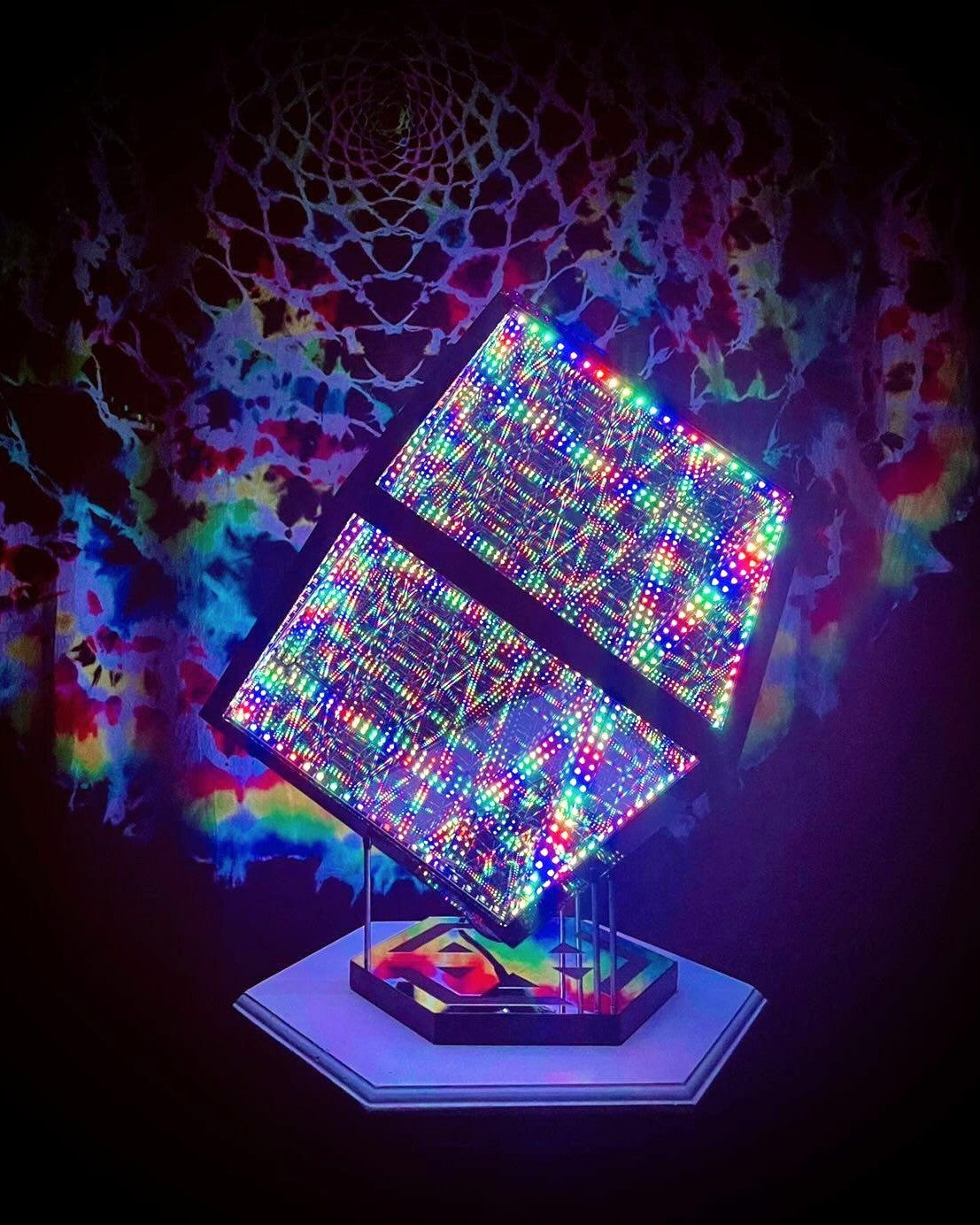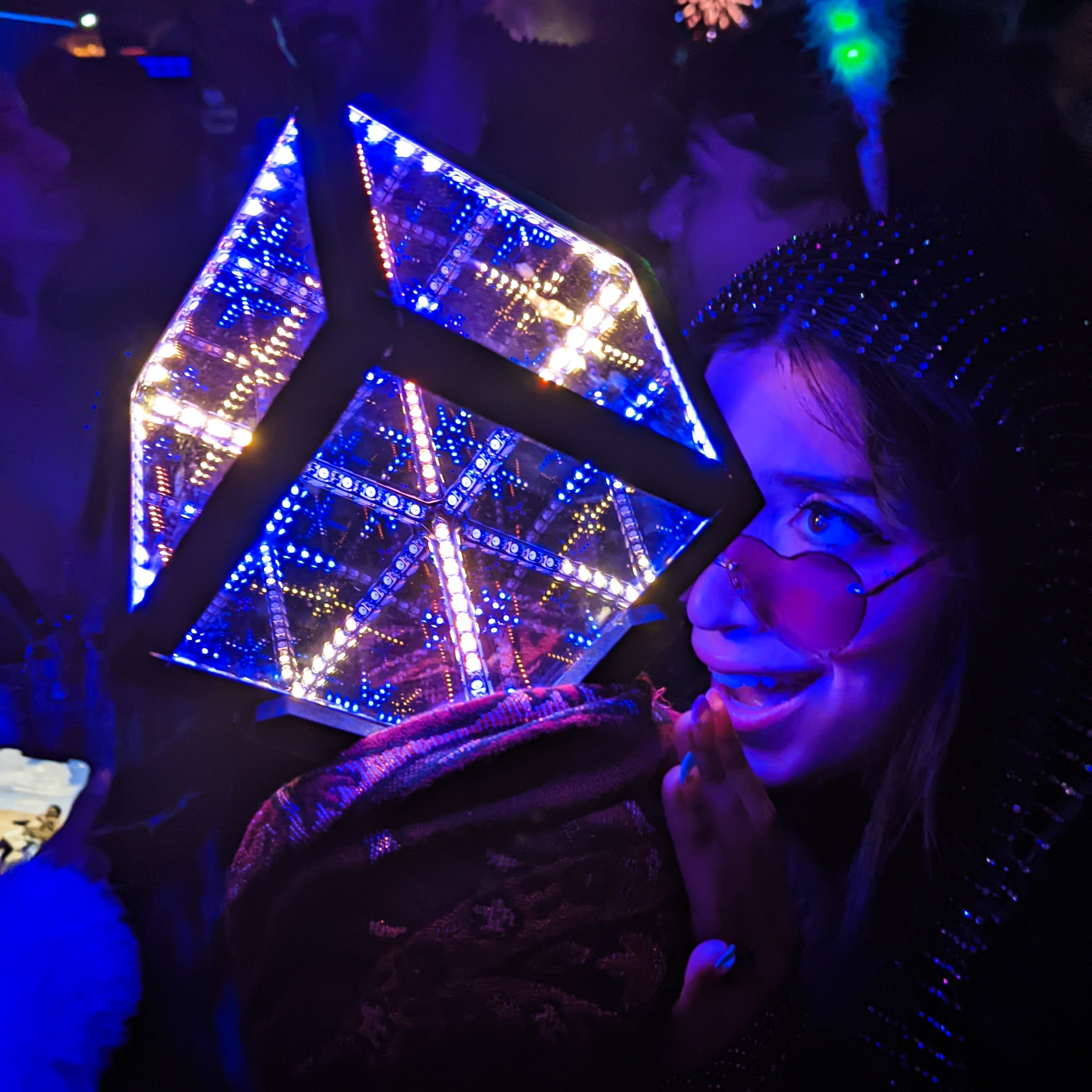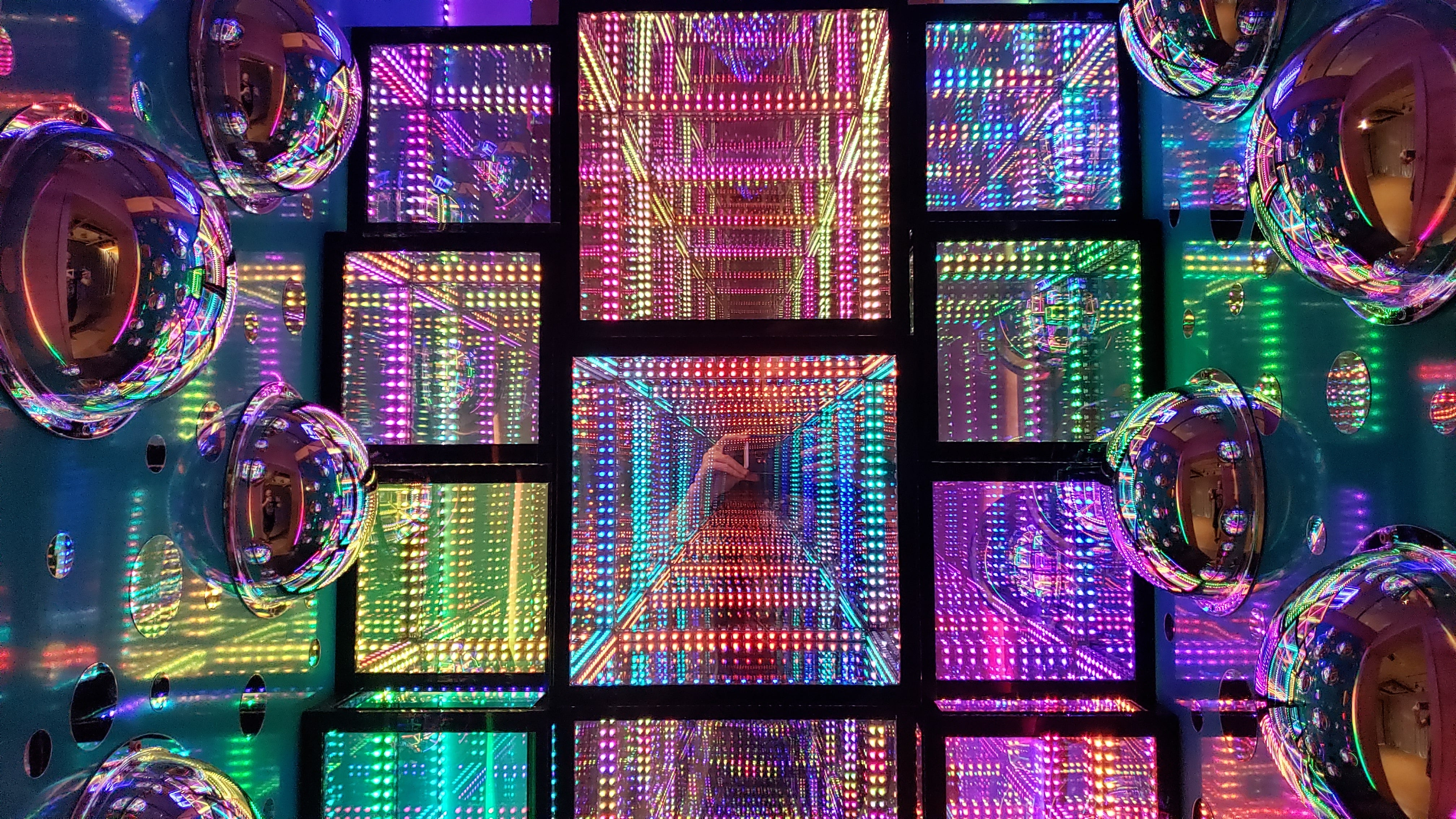# What is an infinite dimensional cube

### Everything You Need to Know About Infinite Dimensional CubesWhat is an infinite dimensional cube? An infinite dimensional cube, also known as the Hilbert cube, is a topological space that is often studied in the field of mathematics. David Hilbert, after whom the cube is named, introduced the concept as a means of demonstrating ideas in topology. The Hilbert cube is defined as the topological product of intervals, making it a cuboid of countably infinite dimensions where the lengths of the edges in each orthogonal direction form a specific sequence. Homeomorphic to the product of countably infinite copies of the unit interval, the Hilbert cube is topologically indistinguishable from the unit cube of countably infinite dimension. It is sometimes referred to as a metric space, and as a subset of a separable Hilbert space, it is often considered without being thought of as a product of copies of the unit interval. If you're studying math at any level, you may encounter the concept of an infinite dimensional cube in your coursework as it provides an important example in topology.### Understanding the Structure of an Infinite Dimensional Cube

An infinite dimensional cube, also known as a hypercube, is a mathematical concept used to represent an n-dimensional cube in a higher-dimensional space. In simpler terms, it is a cube with an infinite number of dimensions. The term "hypercube" was first introduced in the 19th century by mathematician Arthur Cayley. The structure of a hypercube is fascinating and complex, with each additional dimension adding more complexity to its shape. The concept of an infinite dimensional cube is not only relevant to mathematics, but also to art and design. Products inspired by the hypercube, such as the HyperCube10, HyperCube15, and HyperCube Nano from Hyperspace Lighting, showcase its unique structure and offer a futuristic and artistic touch to any space.### Analyzing the Properties of an Infinite Dimensional Cube

What is an infinite dimensional cube, and what makes it unique? An infinite dimensional cube, also known as a hypercube or n-cube, is a mathematical concept that extends the notion of a three-dimensional cube to any number of dimensions. Unlike traditional cubes, which have six faces, eight vertices, and twelve edges, an infinite dimensional cube can have an infinite number of faces, vertices, and edges. It is a complex structure that has fascinated mathematicians and scientists for centuries, and it has also inspired the creation of innovative products like hyper-luminous infinity mirror art pieces that are designed to mimic its intricate properties. Through continued study and analysis, we can gain a better understanding of the properties of the infinite dimensional cube and its potential applications in various fields of science and technology.### Infinite Dimensional Cube: A Journey Through Time and Space

An infinite dimensional cube is a mathematical concept that extends the idea of a 3D cube into higher dimensions. It is a theoretical object that cannot be visualized in the traditional sense, but its properties can be explored and understood through mathematical formulas and equations. The concept of an infinite dimensional cube has applications in fields such as physics, computer science, and topology. One important property of an infinite dimensional cube is that it can be used to represent and analyze complex data sets with high dimensions. This is where products like the HyperCube10 and HyperCube15 come in, which offer hyper-luminous infinity mirror art pieces that can provide a visual representation of an infinite dimensional cube. While the infinite dimensional cube may seem abstract, its applications are vast and significant in many fields of study.### Transform Your Space With An Infinite Dimensional Cube

Transform your space with Hyperspace Lighting's HyperCube10 and HyperCube15, the ultimate infinite dimensional cube art pieces. These cutting-edge fixtures offer a mind-bending optical illusion that will leave your guests in awe. The HyperCube10 and HyperCube15 use the latest technology to provide advanced sound reactivity, customizable app control, and scratch-proof mirrors. Choose from up to 95 unique patterns and 16m+ color combinations to create the perfect ambiance for your space. Elevate your home or office with the HyperCube10 and HyperCube15 from Hyperspace Lighting.

### The Magic of an Infinite Dimensional Cube

An infinite dimensional cube is a fascinating mathematical concept that has intrigued scientists and mathematicians for centuries. At its core, it is a complex structure that can be difficult to visualize. However, its properties are no less extraordinary. The concept of an infinite dimensional cube is based on the idea that as the number of dimensions increases, so too does the number of sides on each of its faces. This creates a structure that is virtually impossible to fully comprehend. But with the HyperCube10 and HyperCube15 infinity mirror art pieces from Hyperspace Lighting, you can experience the magic of an infinite dimensional cube in a whole new way. These innovative light fixtures use cutting-edge technology to create stunning, mesmerizing light shows that are inspired by the infinite dimensional cube. With advanced sound reactivity and customizable app control, the HyperCube10 and HyperCube15 are perfect for anyone who wants to add a touch of futuristic elegance to their space while also experiencing the wonder of an infinite dimensional cube.### Exploring the Fascinating World of Infinite Dimensional Cubes

An infinite dimensional cube is a mathematical concept that goes beyond our basic understanding of space and geometry. It is a theoretical construct that exists in an infinite number of dimensions, with each additional dimension adding another layer of complexity to its structure. While it may seem like an abstract idea, infinite dimensional cubes have real-world applications in fields such as physics, computer science, and even art. Some products utilize the concept of an infinite dimensional cube to create visually stunning pieces, such as HyperCube10 and HyperCube15 infinity mirror art pieces from Hyperspace Lighting. These products allow you to experience the beauty of an infinite dimensional cube firsthand, adding a unique and modern touch to any space. By delving deeper into the world of infinite dimensional cubes, we can gain a greater appreciation for the beauty and complexity of our universe.

### Unlock the Mystery of Infinite Dimensional Cubes

Infinite dimensional cubes are complex mathematical structures that have fascinated mathematicians for centuries. While they may seem abstract and difficult to understand, the concept of infinite dimensions has inspired the creation of stunning visual representations, like the HyperCube10 and HyperCube15, which showcase the beauty of these structures. By exploring the properties and dimensions of infinite dimensional cubes, we gain a deeper understanding of the world around us and the mysteries of the universe. Whether you are a mathematician, an artist, or simply a curious explorer, infinite dimensional cubes offer a fascinating journey through time and space. Start your journey today and unlock the mystery of these fascinating structures.